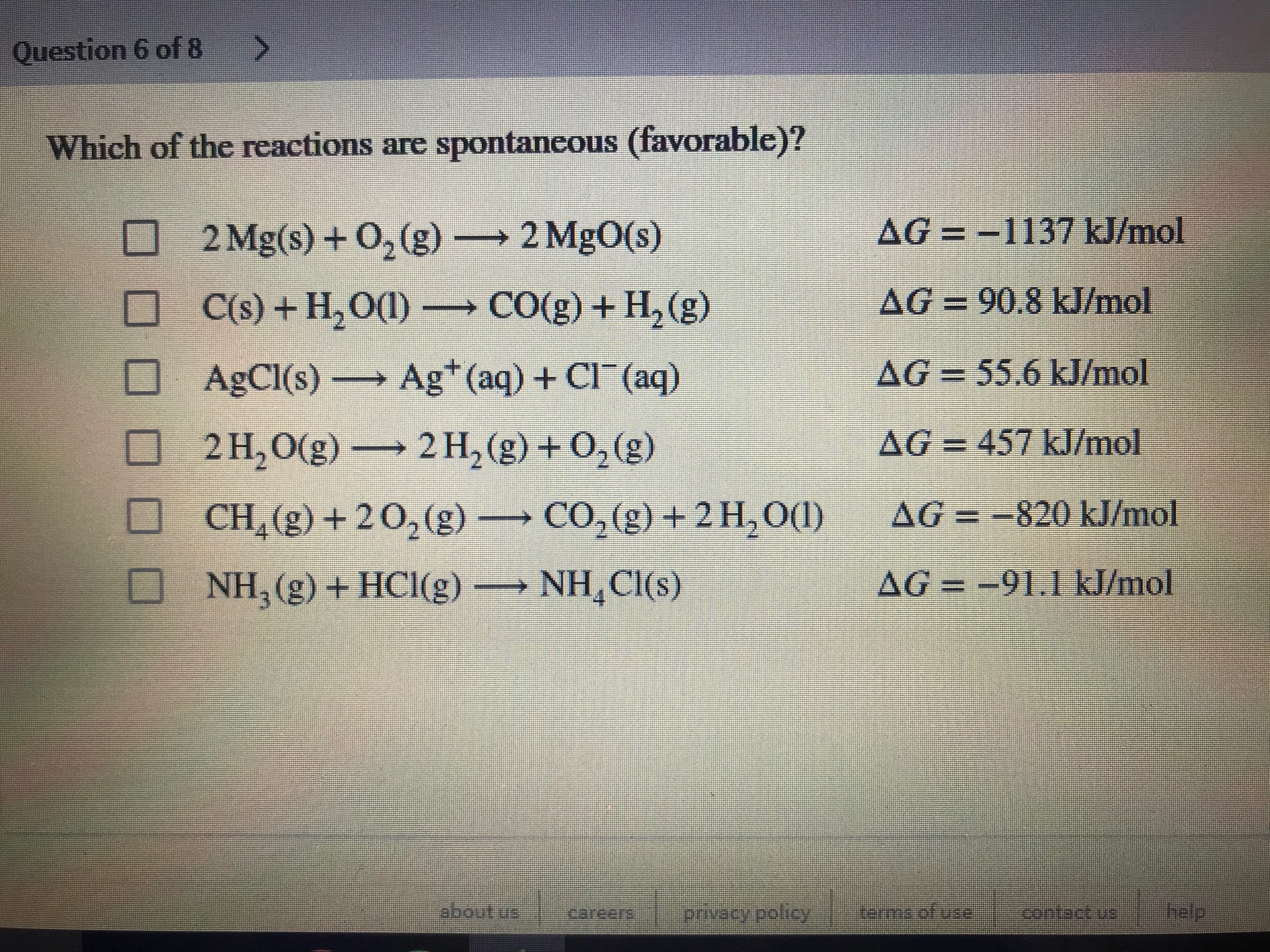# Which of the following reactions are spontaneous (favorable)? a. 2 Mg (s) + O2 → 2MgO (s) ΔG = -1137 kJ/mol b. C(s) + H2O (l) → CO (g) + H2 (g) (s) ΔG = 90.8 kJ/mol c. AgCl (s) → Ag+ (aq) + Cl- (aq) ΔG = 55.6 kJ/mol d. 2H2O→ 2H2 (g) + O2 (g) ΔG = 457 kJ/mol e. CH4 (g) + 2O2 (g) → CO2 (g) + H2O (l) ΔG = -820 kJ/mol f. NH3 (g) + HCl (g) → NH4Cl (aq) ΔG = -91.1 kJ/mol Answer multiple choice please